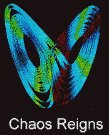## Fractals-a geometry of nature

Fractal geometry plays two roles. It is the geometry of deterministic chaos and it can also describe the geometry of mountains, clouds and galaxies

Benoit Mandelbrot

Science and geometry have always progressed hand in hand. In the 17th century, Johannes Kepler found that he could represent the orbits of the planets around the Sun by ellipses. This stimulated Isaac Newton to explain these elliptical orbits as following from the law of gravity. Similarly, the back-and-forth motion of a perfect pendulum is represented by a sine wave. Simple dynamics used to be associated with simple geometrical shapes. This kind of mathematical picture implies a smooth relationship between an object's form and the forces acting on it. In the examples of the planets and the pendulum, it also implies that the physics is deterministic, meaning that you can predict the future of these systems from their past.
Two recent developments have deeply affected the relationship between geometry and physics, however. The first comes from the recognition that nature is full of something called deterministic chaos. There are many apparently simple physical systems in the Universe that obey deterministic laws but nevertheless behave unpredictably. A pendulum acting under two forces, for example. The notion of deterministic yet unpredictable motion is a surprise to most people.
The second development came from efforts to find mathematical descriptions for some of the most irregular and complicated phenomena we see around us: the shapes of mountains and clouds, how galaxies are distributed in the Universe, and nearer home, the way prices in the financial markets fluctuate. One way of obtaining such a description is to seek a "model". In other words, I had to invent or identify mathematical rules that can produce "mechanical forgeries" of some part of the reality- a photograph of a mountain or a cloud, a map of deepest space,or a chart on the financial pages of a newspaper. Indeed, Galileo proclaimed that "the great book of nature is written in mathematical language", adding that "its characters are triangles, circles and other geometrical figures, without which one wanders in vain through a dark labyrinth". Such Euclidean shapes have, however, proved to be quite useless in modelling either deterministic chaos, or irregular systems. These phenomena need geometries that are very far from triangles and circles. They require non-Euclidean structures in particular, a new geometry called fractal geometry.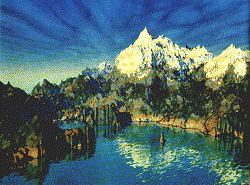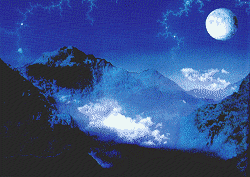Fractal modelling on a computer produces extraordinary landscapes such as these mountain scenes

I coined the word fractal in 1975 from the latin fractus which describes a broken stone-broken up and irregular. Fractals are geometrical shapes that, contrary to those of Euclid, are not regular at all. First, they are irregular all over. Secondly, they have the same degree of irregularity on all scales. A fractal object looks the same when examined from far away or nearby-it is self-similar. As you approach it, however, you find that small pieces of the whole, which seemed from a distance to be formless blobs, become well- defined objects whose shape is roughly that of the previously examined whole.
Nature provides many examples of fractals, for example, ferns, cauliflowers and broccoli, and many other plants, because each branch and twig are very like the whole. The rules governing growth ensure that small-scale features become translated into large-scale ones.

 The Sierpinski gasket- a simple fractal produced by breaking up a triangle into successively smaller ones

A striking mathematical model of the way fractals work is the Sierpinski gasket. Take a black triangle and divide it into four smaller triangles, as shown opposite, and erase the central fourth triangle so that it leaves a white triangle. Each new black triangle will have sides that are half as long as the initial triangle. Repeat the exercise with each new triangle and you obtain the same structure on an ever decreasing scale with a detail that is twice as fine as that in the preceding stage. When parts of the object are exactly like the whole, the object is said to be linearly self-similar.

However, the most important fractals deviate from linear self-similarity. Some of these are fractals that describe general randomness, while others are fractals that can describe chaotic, or nonlinear, systems (where the factors affecting the way the system behaves are not proportional to the effects they produce). Let us take one example of each.
Random fractals became best known through the stream of forgeries of coastlines, mountains and clouds, such as the one on the opposite page, which my colleagues and I have been producing since 1975 using computer graphics. Other examples are some of the scenes made for films such as Star Trek II.
Our work on such fractal modelling began with a bit of folk wisdom and a lot of natural history. The folk wisdom started with observing something that even a cubist painter knows. "Clouds are not spheres, mountains are not cones, coastlines are not circles, and bark is not smooth, nor does lightning travel in a straight line." All these natural structures have irregular shapes that are self-similar. In other words, we discovered that successively magnifying a part of the whole reveals a further structure that is nearly a copy of the original we started with.
The natural history involved collecting and classifying facts about natural structures. For example, as you measure the coast of a country with ever increasing precision, its length becomes greater because you have to take into account ever smaller irregularities along the length. Lewis Fry Richardson has found an empirical law that describes this increase.
To make sense of fractal geometry we have to find ways of expressing the shape and complexity in terms of numbers, just as Euclidean geometry uses the notions of angle, length, area or curvature, and the notions of one, two or three dimensions.
For complicated geometrical objects, the ordinary notion of dimension may vary with scale. As an example, take a ball with a diameter of 10 centimetres made of a thread of 1 millimetre. From far away, the ball appears as a point. From a distance of 10 centimetres, the ball of thread is three- dimensional. At 10 millimetres, it is a mess of one- dimensional threads. At 0.1 millimetres, each thread becomes a column and the whole becomes a three- dimensional object again. At 0.1 millimetres, each column dissolves into fibres, and the ball again becomes one- dimensional, and so on, with the dimension "crossing over" repeatedly from one value to another. When the ball is represented by a finite number of atom-like pinpoints; it becomes zero- dimensional again.Cauliflowers and ferns are examples of natural fractals

For fractals, the counterparts of the familiar dimensions (0, 1,2,3) are known as fractal dimensions. Usually, their values are not whole numbers.
The simplest variant of fractal dimension is the similarity dimension Ds. Applied to a point, a line, a square or a cube, Ds simply gives the number of ordinary dimensions needed to describe the object -0,1,2,3 respectively. What about a curve that is a linearly self-similar fractal? Such a curve can range from being an almost smooth, one-dimensional line to being nearly plane filling, which means that the line twists and turns so much that it visits nearly every part of some region of the plane, becoming almost two-dimensional. Correspondingly the value of Ds, will range up from just above 1 to just below 2. Thus, Ds can be said to measure the complexity of this curve. More generally, Ds measures the complexity or degree of roughness of a fractal shape.
Another simple fractal dimension is the mass dimension. The mass in a one-dimensional straight rod increases in proportion to its length, say, 2R. The mass in a two- dimensional disc of radius R increases in proportion to pR2, the area of a circle. And the mass in a ball increases in proportion to 4/3pR3, the volume of a sphere. So when a further dimension is added, the mass grows in proportion to R raised to a power indicating the number of dimensions. In the case of a fractal, the mass grows proportionately to R raised to some power Dm that is not a whole number. That is, Dm plays one of the usual roles of dimension, so it is natural to call it a fractal dimension. It is fortunate that in all the simple cases, Ds, and Dm (and other definitions of fractal dimension) take precisely the same value. In cases beyond the simplest they may differ.

#### 1: Order and chaos in the Mandelbrot and Julia sets

Take a starting point C0 in the plane of coordinates u0 and v0. From the coordinate of C0 , form a second point C1 of coordinates u1=u02 - v02 + u0 and v1 = 2u0v0 + v0. Next, form the point C2 of coordinates u2 = u12 - v12 + u0 and v2 = 2u1v1 + v0. More generally, the coordinates uk and vk of Ck are obtained from uk - 1 and vk - 1 by the so-called iterative formulas uk = u2k - 1- v2k - 1+ u0 and vk = 2uk - 1vk - 1 + v0.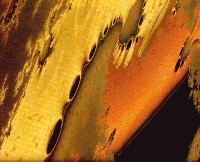The Mandelbrot set produces rich mathematics and beautiful graphics. Here is a general Mandelbrot set, obtained by replacing the usual mathematical formula by a more complicated one.

These formulas may seem a little artificial, but they simplify further if expressed in terms of the mathematics where these formulas originate. A point C of coordinates u and v is represented by the complex number c = u + iv. One can add and multiply complex numbers, except that i2 must always he replaced by - 1. When C0 is represented c0 = u0 + iv, it is easy to verify that the above formulas simply,express that c1 = c02 + c0 and ck = c2k-1 + c0 . Even the reader who is scared of complex numbers will understand the expression in terms of uk and vk.
The points Ck are said to form the orbit of C0 . The Mandelbrot set (M) is defined as follows: if the orbit Ck fails to go to infinity, the point C0 is said to be contained within the set M. If the orbit goes to infinity then the point C0 is outside M.
The original reason for writing this algorithm is that it concerns the problem of the so-called quadratic complex dynamics. When C0 is in the interior of M, the dynamics yields an orbit that is perfectly orderly. When C0 is outside M, the behaviour of the orbit is deterministic but practically unpredictable, or chaotic.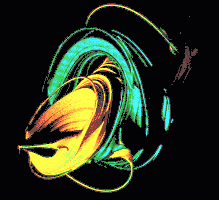Not skeins of coloured knitting wool but a relation of the Mandelbrot set, the Julia set, shown here in four dimensions

The boundary between orderly and chaotic behaviour turns out to he unbelievably messy, so that the set M combines the two themes of order and chaos in more than one way. For example, the illustration on the opposite page represents a zoom upon a tiny piece of M, reduced in ratio of 10-23 to 1. The little "bug" near the centre has very nearly the same shape as the whole Mandelbrot set (this is a token of geometric orderliness). On the other hand, the surrounding pattern is not present in the whole set, but depends very much upon the point on which the zoom has focused (this is a token of variety, and even chaos).
By changing the rules that produce the Mandelbrot set, one obtains a shape called the Julia set. As before, you pick a point C of coordinates u and v, and now you call it a "parameter". Next you pick, in a different plane, a point P0 of coordinates x0 and y0. Then you form x1 = x20 - y20 + u and y1 = 2x0y0 + v. Compared with the rule for the Mandelbrot set, this rule mixes the points (x0, y0) and (u, v). In terms of the complex numbers c = u + iv and z = x + iy, the rule is z1 = z20 + c and (more generally) zk = z2k+ c.
When the orbit Pk fails to escape to infinity, the initial P0 is said to belong to the "filled-in Julia set". To prepare the picture opposite, Alan Norton wept a step further, replacing the complex number z, which is a point in the plane, by a quaternion, which is a point in four-dimensional space. Two difficulties arose: the fourth dimension cannot be plotted, and the Julia set would be so bulky that its structure would be unclear. Therefore, the illustration takes advantage of the fact that there are several "components" to this Julia set, and represents only two of them, in different colours.

The next step in modelling is to imagine the simplest geometrical construction that might have the right properties to generate the structure. In fact, I have put together, and constantly enrich, a toolbox of such constructions that are useful for fractal geometry. To test which mathematical tool would be appropriate, we compare the numerical characteristics of the model with the real thing-the fractal dimensions of a mountain, for example. This is not enough, however. We also use computer graphics to test how good a tool we have.
At the end of the day, we hope to produce a theory from the fractal modelling of mountains that can describe the relief of the Earth.
Because fractals have proved useful in describing complex natural shapes, it is not surprising that fractals also play a part in describing how complex dynamical systems behave. As previous articles in the chaos series have shown, the equations that model turbulence in liquids, the weather, or the dynamics of insect populations are nonlinear and show behaviour typical of deterministic chaos. If we iterate these equations - examine their solutions as they evolve over time - we find that many of the mathematical properties, especially when shown as computer graphics, reveal themselves to be self-similar. Examples are the "phase portraits" of so called strange attractors described last year in Ian Stewart's article (New Scientist, "Portraits of chaos", 4 November 1989).
My best-known contribution to this area of nonlinear fractals is called the Mandelbrot set (see Box 1). The set results from iterating a relatively simple equation. It produces the most extraordinary graphics, rich in complexity. Some people have called it the icon for nonlinear fractal geometry.
The Mandelbrot set does not just produce beautiful pictures. If we examine many pictures with great care, we find innumerable empirical observations that can be restated in the form of mathematical conjectures. Many of these have already led to brilliant theorems and proofs. It has also inspired a new approach to mathematics, using a computer screen.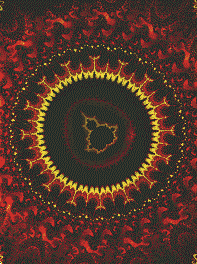The heart of the Mandelbrot set seen here is the result of blowing up the set by Avogadro's number (x 1023). It remains forever intricate on any scale

Mathematical conjectures usually originate in previously known theorems. In recent decades, there was no input at all from physics or from graphics, which meant that some areas of pure mathematics, such as the theory of iteration, (to which the Mandelbrot set belongs) ran out of steam. Fractal pictures done on the computer have revived it. Being able to play with pictures interactively has provided a deep well for mathematical discoveries. Examining the Mandelbrot set has led to many conjectures that were simple to state but hard to prove. Studying them has produced a host of interesting side results.
Of course, many related fractals lead to beautiful and intriguing graphics. Indeed, several shapes known today as fractals were discovered many years ago. Some of these mathematical entities appear in the work of the French group of mathematicians, Henri Poincaré, Pierre Fatou and Gaston Julia, at various times from 1875 to 1925. But nobody realised their significance as visual descriptive tools and their relevance to the physics of the real world.
One model where random fractals describe the real world is a form of random growth called diffusion limited aggregation, or DLA (see below). This yields tree-like shapes of baffling complexity. DLA can be used to model how ash forms, how water seeps through rock,how cracks spread in a solid and how the lightning discharges.
To see how it works, take a very large chess board and put a queen, which is not allowed to move, in the central square. Pawns, which are allowed to move in either of the four directions on the board, are released from a random starting point at the edge of the board, and are instructed to perform a random, or drunkard's walk. The direction of each step is chosen from four equal probabilities. When a pawn reaches a square next to that of the original queen, it transforms itself into a new queen and cannot move any further. Eventually, a branched, rather spidery-looking collection of queens, called a "Witten Sander DLA cluster", grows.
Quite unexpectedly, massive computer simulations have shown that DLA clusters are fractal; they are nearly self- similar. Small portions are very much like reduced versions of large portions. But clusters deviate from randomised linear self-similarity, something that will pose interesting challenges for the future.
What is special about this kind of fractal growth is that it shows very clearly how parameters that vary smoothly produce rugged behaviour. To show how, let us rephrase the original construction in terms of the theory of electrostatic potential. Imagine a big box, in which the DLA is constructed, is set to a positive electrical potential, and the target object, the original queen,is put at the centre and set to a potential of zero. What is the value of the potential elsewhere in the box?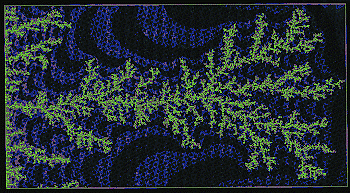A random fractal called a diffusion limited aggregate produces fern-like shapes that model lightning and other natural phenomena

In the cases where the outline of the central object is a smooth curve, or has small number of kinks, like a triangle or a square, scientists have long known how to compute the potential. These classical analytical calculations determine the curves along which the potential is the same. All these curves are smooth, and they provide progressive transitions between the fixed box and the boundary of the fixed object at the centre. Next, suppose that the boundary includes a needle-like projection. Around the needle, the equipotential curves would be very crowded. The drop in potential would be very steep, causing an electrical discharge: the needle would act like a lightning rod. When the centre object is a DLA cluster, all its boundary is lined with needles, and lightning will fall most often on those needles that are the most exposed.
Here comes a critical novelty: the mechanism of DLA is equivalent to postulating that, after a needle has been struck by lightning, it extends or branches. The experiments on DLA teach us when we allow boundaries to move in response to the potential, the cluster grows into an increasingly large DLA structure. This implies we can create rough fractals from the smoothness characteristic of the equation generating the lines of equal potential. Thus, in this context, fractal geometry has led to a new problem and a new area of investigation. Fractal geometry is also being used to describe many other complex phenomena in nature. One of the most fruitful areas is in studying turbulent motion, not only how it arises -the dynamics when displayed as a phase portrait are fractal- but also the complex shapes of turbulent structures. Thus, wakes and jets of water and clouds turn out to be fractal. This must be due to the action of the equations of fluid motion-the Navier- Stokes equations. The problem of relating shape to the dynamics producing it, however, is still wide open. Tracing this relationship would be a major step in understanding turbulence.

#### 2: Multifractal measures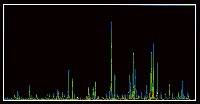Here is a typical trace of multifractals, in this case, modelling turbulence in a laboratory experiment, which has a similar structure to multifractals describing oil distribution

If we take a simple geometrical fractal such as the Sierpinski gasket, we can make some simple and useful mathematical observations. Given a point P in a plane, the question: "Does P belong to the Sierpinski gasket?" has a black-and-white answer, "yes" or "no". Such statements define the gasket as being a fractal set.
Most questions, however, particularly in nature, have a more complicated answer. For example, "Is there any oil in the south of England"? calls for a "many shades of grey" answer, ranging from "bare traces" to "enough to pump even when the price of oil falls very low".
This last answer applies to only a few places, which are unevenly distributed, because they are clustered in few parts of the world. Furthermore, even these parts are mostly barren, so that the distribution of oil is just about as irregular within a country as it is elsewhere in the world. This is an extension of the notion of self-similarity. If we take a line around the globe and plot the availability of oil, we obtain a very wiggly line, with a few sharp peaks separated by large regions of flatness.
How do we represent such an irregular pattern? If we divide the world into regions, each characterised by a different level of oil resources, it happens that each of these regions is nearly a fractal set. So the pattern is not itself a fractal set, but a novel combination of a multitude of fractal sets.It is called a multifractal distribution, or multifractal measure.

Another area where fractals provide an apt description are in living things and in the Universe at large, although in each case, the fractal description breaks down on the very small scale and on the very large. Trees or arteries do not branch endlessly, and whole trees are not part of supertrees.The opposite may be true for the distribution of galaxies in the Universe. Counts of galaxies yield undisputed evidence that at comparatively small scales the distribution is fractal. These small scales are known to extend at least as far as 5 to 10 megaparsecs. There is increasingly strong evidence that there are large voids at a size well above 100 megaparsecs. Such voids are precisely what is expected in a fractal distribution.
How important are fractals? Like for the theory of chaos, it is too early to say for sure, but the prospects are favourable. Many fractals have already had an important cultural impact and have already been accepted as works of a new form of art. Some are representational while others are totally unreal and abstract. It must come as a surprise to both mathematicians and artists to see this kind of cultural interaction.
To the layman, fractal art seems to be magical. But no mathematician can fail to try to understand its structure and its meaning. Much of the underlying equations would have been regarded as part of being pure mathematics, without any application to the real world, had its visual nature not been seen. Most important, as already mentioned, many of the most active uses of fractals are in physics, where they have helped tackle some very old problems and also some altogether new and difficult ones.
A final satisfying spin-off from fractal pictures is that their attractiveness seems to appeal to the young and is having an influence on restoring interest in science. Many people hope that the Mandelbrot set and other fractal pictures, now appearing on T-shirts and posters, will help to give the young a feeling for the beauty and eloquence of mathematics, and its profound relationship with the real world.
 Time fractals in the mind THE incessant background buzz of neurons, once thought to be random like television snow, follows the mathematics of fractals. This finding may alter the way scientists build models of memory. Scientists used to believe that neurotransmitters, chemicals that signal between neurons, were released in random bursts. Now researchers at Boston University and the University of California at San Diego report that the rate of release of neurotransmitters varies in a fractal pattern: it looks similar, regardless of the timescale over which it is observed. Malvin Teich, an electrical engineer at Boston University, teased out single muscle neurons from day-old frog embryos, along with an attached muscle cell. By applying an electrode to the muscle cell, Teich and his colleagues were able to detect the release of single "packets" of neurotransmitter from the tip of the nerve cell. As expected, the frequency at which neurotransmitter packets were released varied constantly. "The rate goes up and down, waxes and wanes,' Teich says. But the variation is not random. If it wem, the fluctuations would average and smooth out over longer time periods. Instead, the average release rate fluctuates as dramatically from minute to minute as from second to second, says Teich in the Journal of Neuroscience (vol 17, p 5666). Such repetition at different scales is a hallmark of fractals. Teich previously found fractal patterns in the hearing and visual systems of cats, but never before in an isolated neuron. This finding means the source of the fractal patterns resides in the nerve cell itself, says Teich. While the function of fractal patterns remains a mystery, Teich says their universal presence suggests they are important. Carl Anderson, a psychobiologist at McLean Hospital, near Boston, agrees. "The most profound aspect of this work is that the events have a correlation over long periods of time." Such long-term correlation, he says, could feature in future models of memory. Jonathan  Knight New Scientist 16 Augiist 1997

 Welcome to fractal country Earth, water and maths explain nature's repeating patterns WHY is nature so keen on fractal designs for its rivers and mountains? Take a look at how water carries soil from place to place and it all becomes perfectly clear, says a geophysicist in New York. Researchers have recognised for some time that the intricate branching structures of river networks are self-similar-that is, their structures appear much alike on very different scales. "If you looked at a river on a map, you wouldn't be able to tell if you were looking at a scale of 1 kilometre or hundreds of kilometres," says Jon Pelletier of Cornell University in Ithaca, New York. This self-similarity, which is the hallmark of fractal systems, is also evident in the land surrounding a river system. The ups and downs of a continent are mirrored by the hills and valleys of a country. Scientists have offered various explanations for why topography should be fractal but none has been completely satisfactory says Pelletier. Now, using an approach that works from the bottom up, Pelletier has come up with a new explanation. "About the only thing we know for sure about the evolution of topography is what happens on a small scale," he says. For example, geologists have a good picture of how fault scarps created by earthquakes change over time. When the ground on one side of a fault is pushed up relative to the ground on the other side, it creates a steep incline with sharp corners at the bottom and top. Over time, as water flows over this scarp, it carries soil from the top edge to the base, rounding both edges. This transformation and other small-scale soil displacements are explained very well by the so-called diffusion equation for sediment transport, says Pelletier. But, he adds, "no one has ever made any connection between this fundamental observation and anything about topography". Pelletier has now combined the diffusion equation with a mathematical description of how the amount of water available to move soil varies with location. In places touched by only a few small streams, little water flows and landscape changes occur slowly. By contrast, in areas where targe rivers run the topography evolves quickly. This interplay in which topography determines water flow and water flow changes topography produces the complicated patterns characteristic of river systems and the land around them, says Pelletier. "You can take this simple model and get very realistic river basins." He has tested his model against seven different places, ranging from river basins in the Himalayas to the Mississippi River Valley and found that its predictions agree quite well with the real thing. Pelletier. who has submitted his results to the Journal of Geophysical Research, says the model also predicts what every hill walker already knows-that land in lower areas tends to be relatively flat compared with land at higher altitudes. "In the low lands you have the largest rivers in the system," he says. And so, on average at least a point in the lowlands will have more water passing over it-and more soil carried off-than points higher up. "Any sort of irregularity in the lowlands topography is not going to last long," Pelletier adds. But variations in the highlands tend to stick around. Of course, even lowlands can be quite hilly in places, Pelletier notes. But in his models the smallest feature is about 100 metres across, and at this scale rivers do keep the low elevations reasonably flat. Robert Pool 12 July 1997 New Scientist

 Author Benoit Mandelbrot is a physicist at the Thomas J. Watson Research Center of IBM, Yorktown Heights, New York, and a mathematician at Yale University. He originated Fractal geometry and has played a major role in developing it. Further Reading Fractal Geometry of Nature, B. B. Mandelbrot. W. H. Freeman, 1982. The Beauty of Fractals, H. O. Peitgen and P. H. Richter, Springer 1986. Fractals,J. Feder, Plenum, 1988.

 Chaos Quantum Logic Cosmos Conscious Belief Elect. Art Chem. Maths

New Scientist 15 September 1990 File Info: Created 8/6/2000 Updated 5/9/2010 Page Address: http://templarseries.fortunecity.com/mandel.html